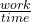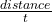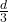## A mover worked 650N. If the mover moved the box in 3 seconds, how many watts of power are used to move the box?

Question

A mover worked 650N. If the mover moved the box in 3 seconds, how many watts of power are used to move the box?

in progress 0
4 months 2021-09-05T15:15:18+00:00 2 Answers 1 views 0

1. 216.6 W,which is the power used to move the box.

Explanation:

Given-

Force,(which is expressed as  F) = 650 N

Time,(which is expressed as  t) = 3 s

Power, P = ?

Formula for Power =Also we can express the P as  F XP = 650P = 216.6 d W

Therefore, 216.6 W ,which is the power  used to move the box.